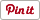Free
Genres:
• Utilities
• Education

Symbolab Calculator app review: helping you solve complex math problems with a few taps 2021

Reviews

Introduction

Symbolab calculator app is a highly sophisticated math calculator that can solve complex problems. This Symbolab algebra calculator app covers advanced mathematics including algebra, calculus, trigonometry, statistics, vectors, and functions and graphing.

The best iPad apps for scientific calculators

The Symbolab step by step app provides the answer to any problem with step by step illustration of each solution. The Symbolab calculator for iPhone also works with the iPad and iPod touch.

For more information on the features and functions of this utility, check out our Symbolab Calculator app review for more details.

Sharpen Your Math Skills with Symbolab Calculator

Advanced math is one of the most difficult subjects in middle school and college. It is not surprising to see many students grapple with numbers to solve problems in algebra, calculus, and advanced vectors and graphing. The good news is that there is now an app that can make advanced mathematics easier.

The Symbolab Calculator is especially designed for students who have trouble finding solutions to complex math problems. This app allows you to enter a specific equation and it will give you the correct the answer in no time.

The best part about Symbolab is that it will give you the step by step solution to the problem with illustrations and graphing, if applicable.

Using the Symbolab app is easy. You can download it for free in the App Store. After installation, you can immediately use the calculator for solving problems.

At the home page, the specialized touch pad with advanced math notations are presented on screen. Specific fields like algebra, calculus, trigonometry, and functions & graphing are located at the top of the screen. So if you are solving algebra problems, tap the algebra section to open this topic.

You can now enter the equation in the calculator and tap ‘Go’ to get the answer. Below the answer is a hidden section called ‘Solution.’

Tap this area to see the step by step process of solving the problem. Most importantly, the app offers links to external sources so you can view more information about the given mathematical concepts.Features of Symbolab Calculator

Symbolab Calculator covers four major advanced math subjects namely, algebra, trigonometry, calculus, and functions and graphing. Each subject covers basic operations as well as advanced equations.

The calculator shows the exact solutions with accurate notations, numerical equivalents, and text explanations. When you use this app, it is like having a personal math tutor in your iOS device.

The application offers graphical representation of solutions, if applicable. This is particularly useful for solving functions and graphing problems, statistical equations, and calculus.

One of the most important features of this app is the step by step solution for every problem. The calculator will show you how each problem has been solved.

By providing the steps to solve complex math problems, the app practically serves as a teaching tool. You can sharpen your problem solving skills by understanding the correct calculation methods.Pros and Cons

Pros

• Offers a simple interface and intuitive workflow
• Covers four major mathematics subjects
• Offers step-by-step solutions with graphs and explanations
• With links to more resources

Cons

• Gets more expensive if you want to unlock advanced featuresFinal Thoughts

Symbolab Calculator is a valuable educational tool for math students. This innovative app can solve the most complex equations with detailed solutions. It is useful for middle schools and college students.